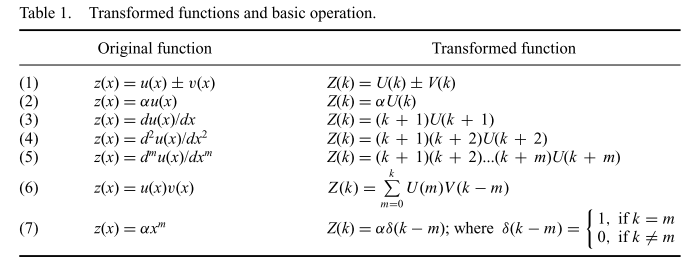Differential transformation method can be used to solve differential equation even integro-differential equations.

Differential transformation is a method that applies the Taylor expansion of the function. For an arbitrary function $f(x)$, we can Taylor expand it

$$\begin{equation} f(x) = \sum_{n=0}^N F(n) (x-x_0)^n, \end{equation}$$

where $F(n)$ is the coefficient of Taylor expansion. By using the definition, we can explore the properties of this transformation from function $f(x)$ to $F(n)$.Table of DTM. From Munganga, J. M. W., Mwambakana, J. N., Maritz, R., Batubenge, T. a., & Moremedi, G. M. (2014). Introduction of the differential transform method to solve differential equations at undergraduate level. International Journal of Mathematical Education in Science and Technology, 45(5), 781–794. http://doi.org/10.1080/0020739X.2013.877609